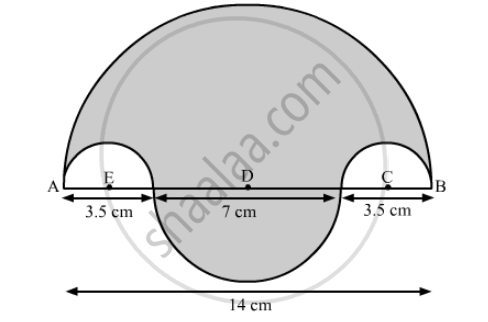Advertisement Remove all ads

# In the Following Figure, the Boundary of the Shaded Region Consists of Four Semi-circular Arcs, the Smallest Two Being Equal. If the Diameter of the Largest is 14 Cm and O - Mathematics

Sum

In the following figure, the boundary of the shaded region consists of four semi-circular arcs, the smallest two being equal. If the diameter of the largest is 14 cm and of the smallest is 3.5 cm, find

the length of the boundary.
the area of the shaded region.Advertisement Remove all ads

#### Solution

We will first find the length of the boundary.

Length of the boundary = perimeter of semi-circle with diameter AB + boundary of semi-circle with diameter 7 cm

"Length of the boundary"=(pixx7+pixx3.5)+pixx1.75+pixx1.75

"Length of the boundary"=pi(7+3.5+1.75+1.75)

"∴Length of the boundary"=14pi

"∴Length of the boundary"=44

Therefore, length of the boundary is44 cm.

Now we will find the area of the shaded region as shown below,

Area of the shaded region = Area of the semi-circle with AB as a diameter − area of the semi-circle with radius AE − area of the semi-circle with radius BC + area of the semi-circle with diameter 7 cm.

∴ "Area of the shaded region"=(pixx7xx7)/2-(pixx1.75xx1.75)/2-(pixx1.75xx1.75)/2+(pixx3.5xx3.5)/2

∴ "Area of the shaded region"=(49pi)/2-3.0625 pi+12.25/2

∴ "Area of the shaded region"=(61.25pi)/2-3.0625pi

∴ "Area of the shaded region"=(61.25pu-6.125pi)/2

∴ "Area of the shaded region"  55.125xx22/7xx1/2

∴ "Area of the shaded region"7.87xx11

∴ "Area of the shaded region"=86.625

Therefore, area of the shaded region is  86.625 cm^2

Is there an error in this question or solution?
Advertisement Remove all ads

#### APPEARS IN

RD Sharma Class 10 Maths
Chapter 13 Areas Related to Circles
Exercise 13.4 | Q 42 | Page 63
Advertisement Remove all ads

#### Video TutorialsVIEW ALL 

Advertisement Remove all ads
Share
Notifications

View all notifications

Forgot password?
Course